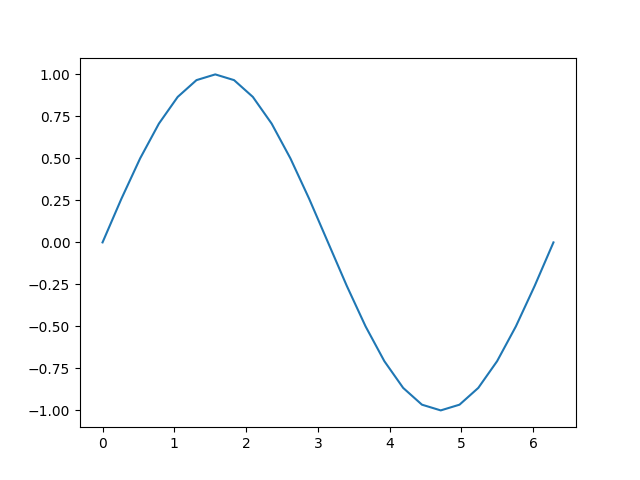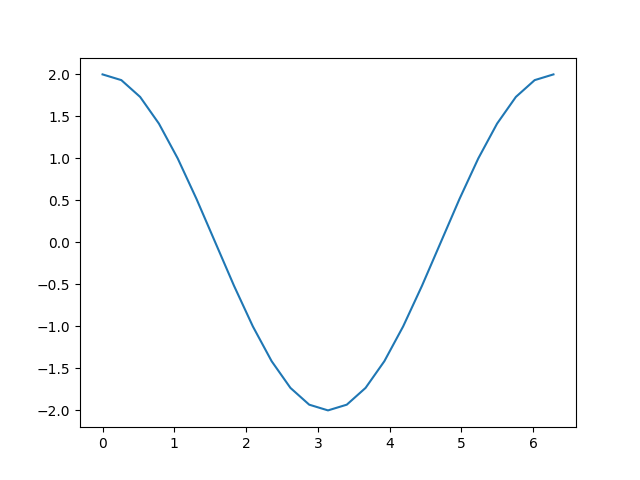# 自动微分的基础知识

PyTorch 的自动微分（ Autograd ）特性使 PyTorch 在构建机器学习项目时更加灵活高效。它能快速简便地计算复杂计算中的多个偏导数（也称为梯度）。这个操作在基于反向传播的神经网络学习中起着核心作用。

## 为什么我们需要自动微分呢？

$$\frac{\partial M(x)}{\partial x}$$ 开始，事情复杂了起来。如果我们再次使用链式法则展开表达式，模型输出关于其输入的偏导数，将涉及到模型中每个乘以学习权重的局部偏导数、每个激活函数以及模型中的其他数学变换。每个局部导数的完整表达式都是计算图中每条 可能路径（欲测量梯度的变量结束的路径）的局部梯度乘积之和。

## 一个简单的例子

# %matplotlib inline

import torch

import matplotlib.pyplot as plt
import matplotlib.ticker as ticker
import math


a = torch.linspace(0., 2. * math.pi, steps=25, requires_grad=True)
print(a)


tensor([0.0000, 0.2618, 0.5236, 0.7854, 1.0472, 1.3090, 1.5708, 1.8326, 2.0944,
2.3562, 2.6180, 2.8798, 3.1416, 3.4034, 3.6652, 3.9270, 4.1888, 4.4506,
4.7124, 4.9742, 5.2360, 5.4978, 5.7596, 6.0214, 6.2832],


b = torch.sin(a)
plt.plot(a.detach(), b.detach())[<matplotlib.lines.Line2D object at 0x7f598d0477c0>]


print(b)


tensor([ 0.0000e+00,  2.5882e-01,  5.0000e-01,  7.0711e-01,  8.6603e-01,
9.6593e-01,  1.0000e+00,  9.6593e-01,  8.6603e-01,  7.0711e-01,
5.0000e-01,  2.5882e-01, -8.7423e-08, -2.5882e-01, -5.0000e-01,
-7.0711e-01, -8.6603e-01, -9.6593e-01, -1.0000e+00, -9.6593e-01,
-8.6603e-01, -7.0711e-01, -5.0000e-01, -2.5882e-01,  1.7485e-07],


c = 2 * b
print(c)

d = c + 1
print(d)


tensor([ 0.0000e+00,  5.1764e-01,  1.0000e+00,  1.4142e+00,  1.7321e+00,
1.9319e+00,  2.0000e+00,  1.9319e+00,  1.7321e+00,  1.4142e+00,
1.0000e+00,  5.1764e-01, -1.7485e-07, -5.1764e-01, -1.0000e+00,
-1.4142e+00, -1.7321e+00, -1.9319e+00, -2.0000e+00, -1.9319e+00,
-1.7321e+00, -1.4142e+00, -1.0000e+00, -5.1764e-01,  3.4969e-07],
tensor([ 1.0000e+00,  1.5176e+00,  2.0000e+00,  2.4142e+00,  2.7321e+00,
2.9319e+00,  3.0000e+00,  2.9319e+00,  2.7321e+00,  2.4142e+00,
2.0000e+00,  1.5176e+00,  1.0000e+00,  4.8236e-01, -3.5763e-07,
-4.1421e-01, -7.3205e-01, -9.3185e-01, -1.0000e+00, -9.3185e-01,
-7.3205e-01, -4.1421e-01,  4.7684e-07,  4.8236e-01,  1.0000e+00],


out = d.sum()
print(out)


print('d:')
print('\nc:')
print('\nb:')
print('\na:')


d:
((<MulBackward0 object at 0x7f598d4ad450>, 0), (None, 0))
((<SinBackward0 object at 0x7f598d4ad450>, 0), (None, 0))
()

c:

b:

a:
None


out.backward()tensor([ 2.0000e+00,  1.9319e+00,  1.7321e+00,  1.4142e+00,  1.0000e+00,
5.1764e-01, -8.7423e-08, -5.1764e-01, -1.0000e+00, -1.4142e+00,
-1.7321e+00, -1.9319e+00, -2.0000e+00, -1.9319e+00, -1.7321e+00,
-1.4142e+00, -1.0000e+00, -5.1764e-01,  2.3850e-08,  5.1764e-01,
1.0000e+00,  1.4142e+00,  1.7321e+00,  1.9319e+00,  2.0000e+00])

[<matplotlib.lines.Line2D object at 0x7f598d487070>]


a = torch.linspace(0., 2. * math.pi, steps=25, requires_grad=True)
b = torch.sin(a)
c = 2 * b
d = c + 1
out = d.sum()


## 训练中的自动微分

BATCH_SIZE = 16
DIM_IN = 1000
HIDDEN_SIZE = 100
DIM_OUT = 10

class TinyModel(torch.nn.Module):

def __init__(self):
super(TinyModel, self).__init__()

self.layer1 = torch.nn.Linear(1000, 100)
self.relu = torch.nn.ReLU()
self.layer2 = torch.nn.Linear(100, 10)

def forward(self, x):
x = self.layer1(x)
x = self.relu(x)
x = self.layer2(x)
return x

model = TinyModel()


print(model.layer2.weight[0:10]) # just a small slice


tensor([ 0.0920,  0.0916,  0.0121,  0.0083, -0.0055,  0.0367,  0.0221, -0.0276,
None


optimizer = torch.optim.SGD(model.parameters(), lr=0.001)

prediction = model(some_input)

loss = (ideal_output - prediction).pow(2).sum()
print(loss)


tensor(211.2634, grad_fn=<SumBackward0>)


loss.backward()
print(model.layer2.weight[0:10])


tensor([ 0.0920,  0.0916,  0.0121,  0.0083, -0.0055,  0.0367,  0.0221, -0.0276,
tensor([12.8997,  2.9572,  2.3021,  1.8887,  5.0710,  7.3192,  3.5169,  2.4319,
0.1732, -5.3835])


optimizer.step()
print(model.layer2.weight[0:10])


tensor([ 0.0791,  0.0886,  0.0098,  0.0064, -0.0106,  0.0293,  0.0186, -0.0300,
tensor([12.8997,  2.9572,  2.3021,  1.8887,  5.0710,  7.3192,  3.5169,  2.4319,
0.1732, -5.3835])


print(model.layer2.weight.grad[0:10])

for i in range(0, 5):
prediction = model(some_input)
loss = (ideal_output - prediction).pow(2).sum()
loss.backward()



tensor([12.8997,  2.9572,  2.3021,  1.8887,  5.0710,  7.3192,  3.5169,  2.4319,
0.1732, -5.3835])
tensor([ 19.2095, -15.9459,   8.3306,  11.5096,   9.5471,   0.5391,  -0.3370,
8.6386,  -2.5141, -30.1419])
tensor([0., 0., 0., 0., 0., 0., 0., 0., 0., 0.])


## 启停自动微分

a = torch.ones(2, 3, requires_grad=True)
print(a)

b1 = 2 * a
print(b1)

b2 = 2 * a
print(b2)


tensor([[1., 1., 1.],
tensor([[2., 2., 2.],
tensor([[2., 2., 2.],
[2., 2., 2.]])


a = torch.ones(2, 3, requires_grad=True) * 2
b = torch.ones(2, 3, requires_grad=True) * 3

c1 = a + b
print(c1)

c2 = a + b

print(c2)

c3 = a * b
print(c3)


tensor([[5., 5., 5.],
tensor([[5., 5., 5.],
[5., 5., 5.]])
tensor([[6., 6., 6.],


torch.no_grad() 也可以用作函数或方法装饰器：

def add_tensors1(x, y):
return x + y

return x + y

a = torch.ones(2, 3, requires_grad=True) * 2
b = torch.ones(2, 3, requires_grad=True) * 3

print(c1)

print(c2)


tensor([[5., 5., 5.],
tensor([[5., 5., 5.],
[5., 5., 5.]])


x = torch.rand(5, requires_grad=True)
y = x.detach()

print(x)
print(y)


tensor([0.0670, 0.3890, 0.7264, 0.3559, 0.6584], requires_grad=True)
tensor([0.0670, 0.3890, 0.7264, 0.3559, 0.6584])


### 自动微分和原地操作

a = torch.linspace(0., 2. * math.pi, steps=25, requires_grad=True)
torch.sin_(a)


## 自动微分分析器

device = torch.device('cpu')
run_on_gpu = False
if torch.cuda.is_available():
device = torch.device('cuda')
run_on_gpu = True

for _ in range(1000):
z = (z / x) * y

print(prf.key_averages().table(sort_by='self_cpu_time_total'))


-------------------------  ------------  ------------  ------------  ------------  ------------  ------------  ------------  ------------  ------------  ------------
Name    Self CPU %      Self CPU   CPU total %     CPU total  CPU time avg     Self CUDA   Self CUDA %    CUDA total  CUDA time avg    # of Calls
-------------------------  ------------  ------------  ------------  ------------  ------------  ------------  ------------  ------------  ------------  ------------
aten::div        51.16%       6.308ms        51.16%       6.308ms       6.308us      16.511ms        50.67%      16.511ms      16.511us          1000
aten::mul        48.67%       6.001ms        48.67%       6.001ms       6.001us      16.072ms        49.33%      16.072ms      16.072us          1000
cudaDeviceSynchronize         0.18%      22.000us         0.18%      22.000us      22.000us       0.000us         0.00%       0.000us       0.000us             1
-------------------------  ------------  ------------  ------------  ------------  ------------  ------------  ------------  ------------  ------------  ------------
Self CPU time total: 12.331ms
Self CUDA time total: 32.583ms


## 进阶主题：更多的自动微分细节和高级 API

$J=\begin{pmatrix} \frac{\partial y_1}{\partial x_1} & \cdots & \frac{\partial y_1}{\partial x_n} \\ \vdots & \ddots & \vdots \\ \frac{\partial y_m}{\partial x_1} & \cdots & \frac{\partial y_m}{\partial x_n} \end{pmatrix}$

$J^T \cdot b=\begin{pmatrix} \frac{\partial y_1}{\partial x_1} & \cdots & \frac{\partial y_m}{\partial x_1} \\ \vdots & \ddots & \vdots \\ \frac{\partial y_1}{\partial x_n} & \cdots & \frac{\partial y_m}{\partial x_n} \end{pmatrix} \begin{pmatrix} \frac{\partial l}{\partial y_1} \\ \vdots \\ \frac{\partial l}{\partial y_m} \\ \end{pmatrix} = \begin{pmatrix} \frac{\partial l}{\partial x_1} \\ \vdots \\ \frac{\partial l}{\partial x_n} \\ \end{pmatrix}$

torch.autograd 是计算这些乘积的引擎，我们就是这样在后向传递过程中累积学习权重的梯度的。

x = torch.randn(3, requires_grad=True)

y = x * 2
while y.data.norm() < 1000:
y = y * 2

print(y)


tensor([  299.4868,   425.4009, -1082.9885], grad_fn=<MulBackward0>)


v = torch.tensor([0.1, 1.0, 0.0001], dtype=torch.float) # stand-in for gradients
y.backward(v)



tensor([1.0240e+02, 1.0240e+03, 1.0240e-01])


（请注意，输出梯度都与 2 的幂次有关——这与重复的倍增操作是一致的。）

### 高级 API

def exp_adder(x, y):
return 2 * x.exp() + 3 * y

inputs = (torch.rand(1), torch.rand(1)) # arguments for the function
print(inputs)


(tensor([0.7212]), tensor([0.2079]))

(tensor([[4.1137]]), tensor([[3.]]))


inputs = (torch.rand(3), torch.rand(3)) # arguments for the function
print(inputs)


(tensor([0.2080, 0.2604, 0.4415]), tensor([0.5220, 0.9867, 0.4288]))

(tensor([[2.4623, 0.0000, 0.0000],
[0.0000, 2.5950, 0.0000],
[0.0000, 0.0000, 3.1102]]), tensor([[3., 0., 0.],
[0., 3., 0.],
[0., 0., 3.]]))


torch.autograd.functional.hessian()方法的工作原理与此相同（假设函数是二次微分的），但返回的是所有二阶导数的矩阵。

def do_some_doubling(x):
y = x * 2
while y.data.norm() < 1000:
y = y * 2
return y

inputs = torch.randn(3)

(tensor([-665.7186, -866.7054,  -58.4194]), tensor([1.0240e+02, 1.0240e+03, 1.0240e-01]))

torch.autograd.functional.jvp() 方法执行与 vjp() 相同的矩阵乘法，但操作数相反。vhp()hvp() 方法也是对向量和 Hessian 乘积执行相同的操作。